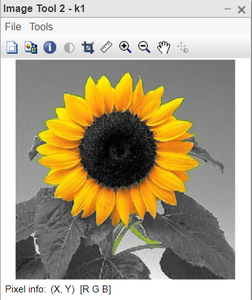Open In App

# Change background of color image into grayscale in MATLAB

In this article, we will discuss how to change the background of a colour image into grayscale using MATLAB. We are focused on the RGB colour model. An RGB colour image is taken. The entire image is converted into grayscale except for the object highlighted in the image. We kept the colour of the flower yellow and converted the rest of the background into Grayscale.

### Utility Function:

This is the manually written function. It converts the colour image into grayscale first of all. A new colour image is created and all three channels (RGB) are assigned the values of the grayscale image(obtained in the first step). The idea is to keep the colour of the interesting portion of the image and keep the rest of the pixels as grayscale. We have noticed that for the central flower part, the Red intensity values are greater than equal to 110; the Green intensity values are greater than equal to 70 and the Blue intensity values are less than 90.

Upon completing the traversing and modifying the newly created image. We return the new image.

### Approach:

• Reading images: First, the input image is read and stored in a variable named K. Images are read into the MATLAB Environment using imread() function which takes filename with applicable extension as the argument. The given below syntax read JPEG image into the image array.
`I=imread('image.jpg');`
• Image is passed to utility function for modification.
• Showing images: Original and modified images are displayed. Images are shown into the MATLAB environment using the imtool() function. imtool opens a new Image Tool in an empty state. We can use the File menu options Open or Import from Workspace to choose an image for display.
`imtool(k1,[]);`
• Utility function sets all 3 channel pixel intensities for the central flower part.
• Utility function sets all 3 channels pixel intensities the same for the rest of the background equal to a grayscale image.

Now, we have taken an example to change the background colour of an image.

Example:

## Matlab

 `% MATLAB code for Change background to GRAY``% Read image and pass to function.``k=imread(``"yellow2.jpeg"``);``imtool(k,[]);``k1=changeBG(k);``imtool(k1,[]);`` ` `% Utility function.``function` `f=changeBG(img)``k=rgb2gray(img);``k1(:,:,1)=k;``k1(:,:,2)=k;``k1(:,:,3)=k;``[x,y]=size(k);``for` `i=1:x``    ``for` `j=1:y``        ``if``(img(i,j,1)>=110 && img(i,j,2)>=70 && img(i,j,3)<90)``            ``k1(i,j,1)=img(i,j,1);``            ``k1(i,j,2)=img(i,j,2);``            ``k1(i,j,3)=img(i,j,3);``        ``end``    ``end``end``f=k1;``end`

Output:Figure: Original Colour imageFigure: Gray Background image